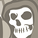# QlikView App Dev

Discussion Board for collaboration related to QlikView App Development.

Announcements
QlikWorld 2022, LIVE in Denver CO., May 16-19, 2022. REGISTER NOW TO RECEIVE EARLY BIRD PRICING
cancel
Showing results for
Did you mean:Contributor II

## Previous period in one column with current (pivot table)

I am wondering, it is possible to get previous period sum in one column with current period?

for ex. now, i have table like this:I use  this formula for calculating last period

=SUM({\$< Field1 = {'Income'} ,  Period = {"\$(=

)"}>}  Value)

1 Solution

Accepted SolutionsMVP

Try this

Before(Sum({\$<Field1 = {'Income'}, Period = {"\$(='>=' & Num(AddYears(Min(Period), -1)) & '<=' & Num(AddYears(Max(Период), -1)))"}>} Value))

Missing Manual - Before() and After()

3 RepliesMVP

Try this

Before(Sum({\$<Field1 = {'Income'}, Period = {"\$(='>=' & Num(AddYears(Min(Period), -1)) & '<=' & Num(AddYears(Max(Период), -1)))"}>} Value))

Missing Manual - Before() and After()Master

use another Dimension than year maybe?Contributor II
Author

Sunny, thx =). It's helpedCommunity Browser